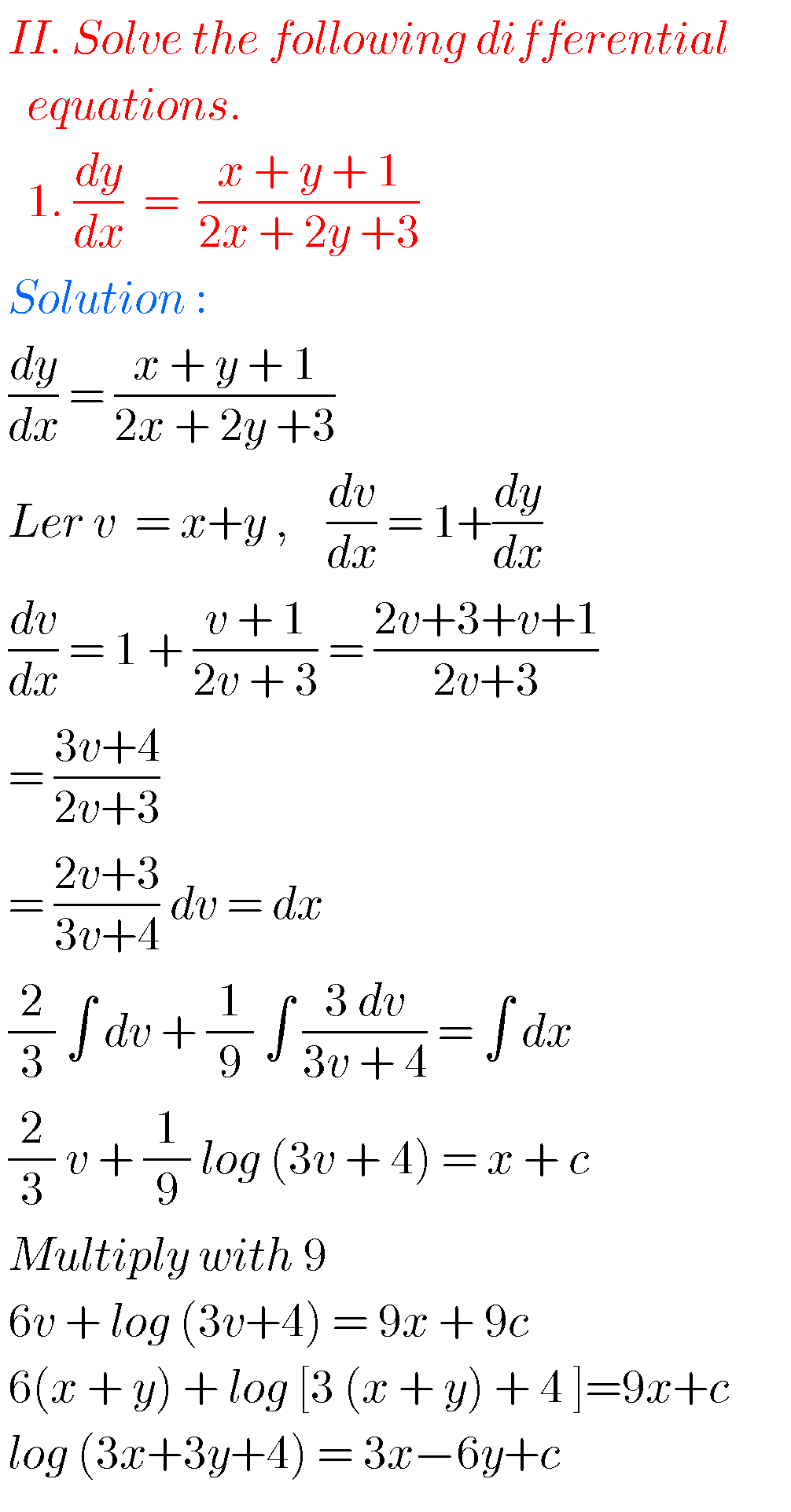## Intermediate Maths solutions for Differential Equations

Intermediate Maths solutions for Differential Equations Inter Maths IIB solutions for Differential Equations exercise 8(a), 8(b), 8(c), 8(d) and 8(e) are given. These solutions are very easy to understand. First you study the text book lesson ver well. Observe the example problems and solutions. Try them. Observe the given solutions and try them in your …